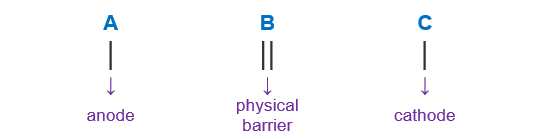# Problem: Consider the following half-reactions and their standard reduction potentials then give the standard line (cell) notation for a voltaic cell built on these half reactions.Mn2+(aq) + 2 e- &lt;=&gt; Mn(s) E° =  -1.18 VFe3+(aq) + 3 e- &lt;=&gt; Fe(s) E° =  -0.036 Va. Mn2+(aq, 1.0 M) | Mn(s) || Fe(s) | Fe3+(aq, 1.0 M)b. Mn(s) | Mn2+(aq, 1.0 M) || Fe3+(aq, 1.0 M) | Fe(s)c. Fe3+(aq, 1.0 M) | Fe(s) || Mn(s) | Mn2+(aq, 1.0 M)d.  Fe(s) | Fe3+(aq, 1.0 M) ||  Mn2+(aq, 1.0 M) | Mn(s)e.  Mn2+(aq, 1.0 M) | Fe3+(aq, 1.0 M) ||  Fe(s) | Mn(s)

###### FREE Expert Solution

We’re being asked to give the standard line (cell) notation for a voltaic cell built on these half reactions below:

Mn2+(aq) + 2 e- ⇌ Mn(s)           E° =  -1.18 V
Fe3+(aq) + 3 e- ⇌ Fe(s)             E° =  -0.036 V

When writing a cell notation, we use the following format – “as easy as ABCTo determine the cell notation of the reaction, we will use the following steps:

Step 1: Determine the anode and the cathode.
Step 2: Write the cell notation of the reaction.

92% (21 ratings)###### Problem Details

Consider the following half-reactions and their standard reduction potentials then give the standard line (cell) notation for a voltaic cell built on these half reactions.

Mn2+(aq) + 2 e- <=> Mn(s) E° =  -1.18 V

Fe3+(aq) + 3 e- <=> Fe(s) E° =  -0.036 V

a. Mn2+(aq, 1.0 M) | Mn(s) || Fe(s) | Fe3+(aq, 1.0 M)
b. Mn(s) | Mn2+(aq, 1.0 M) || Fe3+(aq, 1.0 M) | Fe(s)
c. Fe3+(aq, 1.0 M) | Fe(s) || Mn(s) | Mn2+(aq, 1.0 M)
d.  Fe(s) | Fe3+(aq, 1.0 M) ||  Mn2+(aq, 1.0 M) | Mn(s)
e.  Mn2+(aq, 1.0 M) | Fe3+(aq, 1.0 M) ||  Fe(s) | Mn(s)

Frequently Asked Questions

What scientific concept do you need to know in order to solve this problem?

Our tutors have indicated that to solve this problem you will need to apply the Cell Notation concept. If you need more Cell Notation practice, you can also practice Cell Notation practice problems.